﻿ 基于曲波变换的航空电磁数据去噪方法研究
 地球物理学报2020, Vol. 63Issue (12): 4592-4603PDF

1. 吉林大学地球探测科学与技术学院, 长春 130026;
2. 桂林理工大学地球科学学院, 桂林 541006

Airborne EM denoising based on curvelet transform
WANG Ning1, YIN ChangChun1, GAO LingQi1, SU Yang1, LIU YunHe1, XIONG Bin2
1. College of Geo-Exploration Science and Technology, Jilin University, Changchun 130026, China;
2. College of Earth Sciences, Guilin University of Technology, Guilin 541006, China
Abstract: The airborne electromagnetic (AEM) method has become an effective tool for exploration in areas with complex topography and is widely applied in the world. However, most current AEM systems are mounted or towed in a dynamic environment, resulting in big noise interference. To improve the quality of AEM data and interpretation, the denoising technique needs to be developed. Traditional denoising methods work mostly on special noise or single survey lines, without taking into account the correlation of signal at neighboring survey lines. In this work, we develop a denoising technique based on the curvelet transform for AEM data. As curvelet transform has the characteristics of multiple-scale and multiple-direction, it can remove the noise based on detailed analysis to the signal, while at the same time it considers the signal correlation between neighboring survey lines. Further, we improve the quality of denoising results by introducing the Sigmoid threshold function. We test our method by denoising both synthetic and survey data. The numerical results show that the curvelet-based method has obvious advantage in denoising AEM data.
Keywords: Airborne EM    Curvelet transform    Denoising    Multi-scale analysis
0 引言

1 曲波变换及去噪理论

1.1 波数域内的窗函数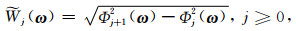(1)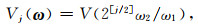(2)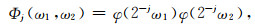(3)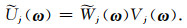(4)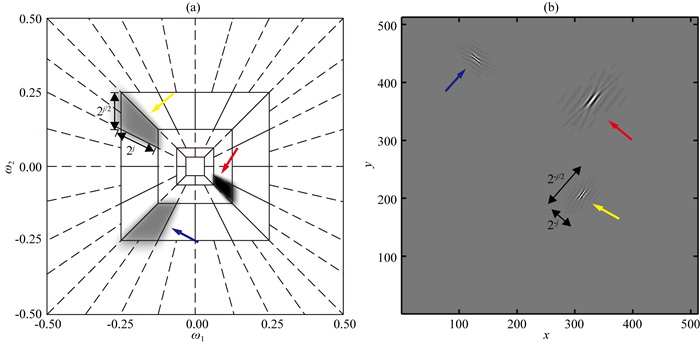图 1 (a) 波数域中的楔形窗；(b)与波数域中楔形窗对应的空间域曲波(参考Herrmann and Hennenfent, 2008) Fig. 1 (a) Wedges in wavenumber domain; (b) Curvelets in spatial domain corresponding to wedges in the wavenumber domain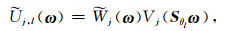(5)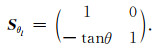(6)

1.2 空间域内的曲波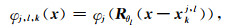(7)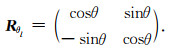(8)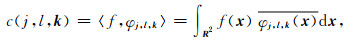(9)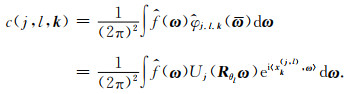(10)

1.3 基于Wrapping技术的离散曲波变换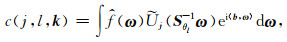(11)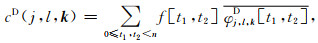(12)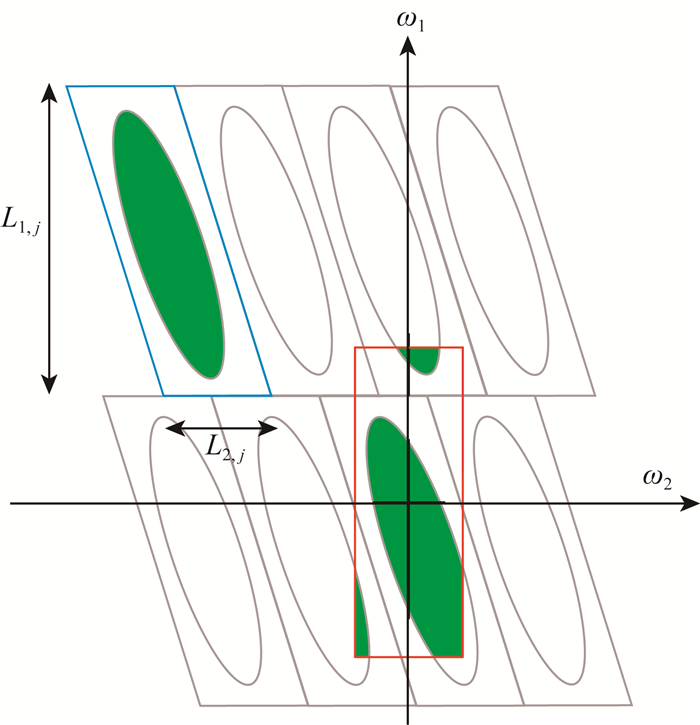图 2 曲波变换中的Wrapping技术 蓝色平行四边形为波数域中的一个支撑区间，绿色区域包含该尺度和方向内的信息，Wrapping过程将该区域内的信息转化到围绕原点的红色矩形支撑区间内(参考Candès et al., 2006). Fig. 2 Wrapping process in curvelet transform The blue parallelogram is a support interval in wavenumber domain and the green area contains the information in this scale and direction. The wrapping process transfers the information to the red rectangular around the origin.

Wrapping技术的核心步骤是围绕原点做包络，目的在于将所有区域都通过平移映射到位于原点的矩形仿射区域，由于二维傅里叶变换具有周期性，故此映射过程不对信息造成损失.经过此步骤，傅里叶变换得以实现.

1.4 曲波域中信号特征分析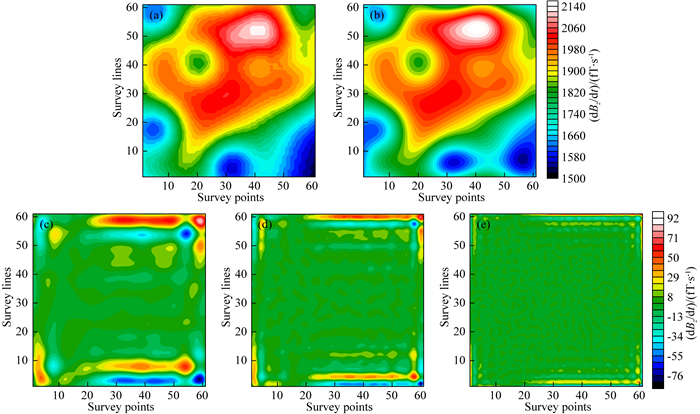图 3 理论磁感应强度时间导数信号及其在不同尺度上的重构结果 (a)理论信号；(b)粗尺度重构结果；(c)第二尺度重构结果；(d)第三尺度重构结果；(e)第四尺度重构结果. Fig. 3 Theoretical magnetic induction and its reconstruction results at different scales (a) Theoretical signal; (b) The coarse scale reconstruction result; (c) The 2nd scale reconstruction result; (d) The 3rd scale reconstruction result; (e) The 4th scale reconstruction result.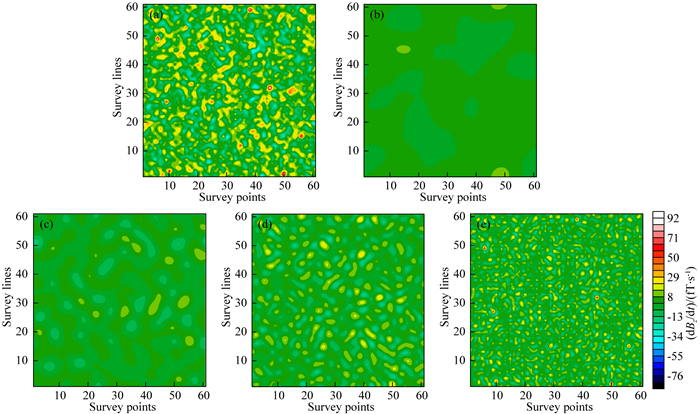图 4 全随机磁感应强度时间导数(噪声)及其在不同尺度上的重构结果 (a)噪声；(b)粗尺度重构结果；(c)第二尺度重构结果；(d)第三尺度重构结果；(e)第四尺度重构结果. Fig. 4 Random noise and its reconstruction results at different scales (a) Noise; (b) The coarse scale reconstruction result; (c) The 2nd scale reconstruction result; (d) The 3rd scale reconstruction result; (e) The 4th scale reconstruction result.

1.5 阈值函数设置

(1) 硬阈值函数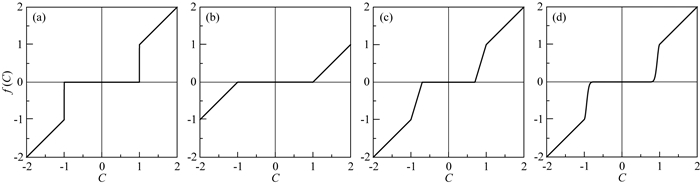图 5 (a) 硬阈值函数(T=1); (b)软阈值函数(T=1); (c)线性阈值函数(T1=0.8，T2=1); (d)非线性阈值函数(T1=0.8，T2=1) Fig. 5 (a) Hard threshold function (T=1); (b) Soft threshold function (T=1); (c) Linear threshold function (T1=0.8, T2=1); (d) Nonlinear threshold function (T1=0.8, T2=1)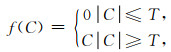(13)

(2) 软阈值函数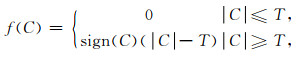(14)

(3) 线性阈值函数和非线性阈值函数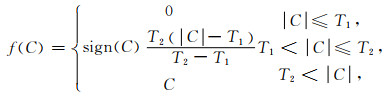(15)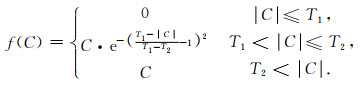(16)

(4) Sigmoid阈值函数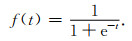(17)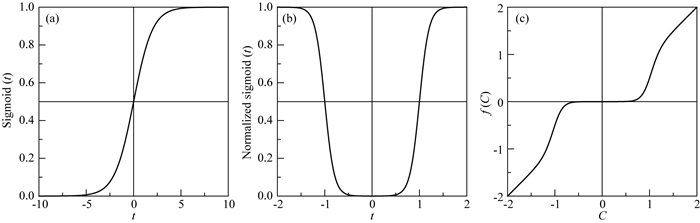图 6 (a) Sigmoid函数；(b)归一化后的Sigmoid函数(T=1)；(c) Sigmoid阈值函数(T=1) Fig. 6 (a) Sigmoid function; (b) Sigmoid function after normalizing (T=1); (c) Sigmoid threshold function (T=1)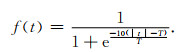(18)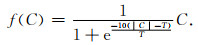(19)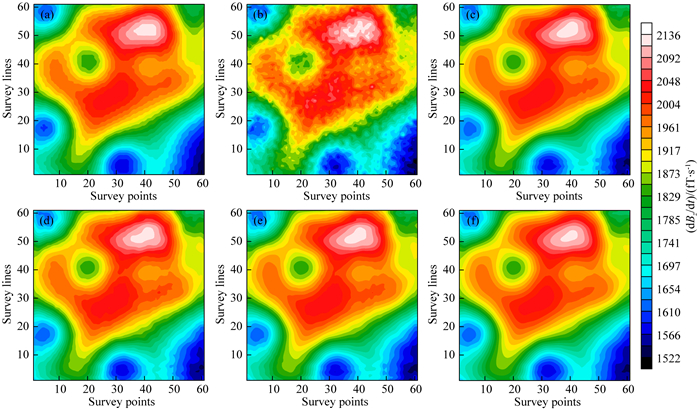图 7 (a) 图 3a中给出的理论信号；(b) 图 3a中的理论信号和图 4a中的随机噪声的叠加结果，SNR=39.61 dB；(c)硬阈值函数去噪，SNR=47.35 dB；(d)软阈值函数去噪，SNR=46.83 dB；(e)非线性阈值函数去噪，SNR=47.47 dB；(f) Sigmoid阈值函数去噪，SNR=48.72 dB Fig. 7 (a) Theoretical signal in Fig. 3a; (b) Addition of the theoretical signal in Fig. 3a and the random noise in Fig. 4a, SNR=39.61 dB; (c) Result of hard threshold function denoising, SNR=47.35 dB; (d) Result of soft threshold function denoising, SNR=46.83 dB; (e) Result of nonlinear threshold function denoising, SNR=47.47 dB; (f) Result of Sigmoid threshold function denoising, SNR=48.72 dB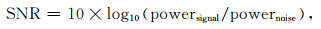(20)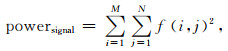(21)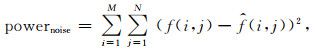(22)

2 数值试验 2.1 理论模型试验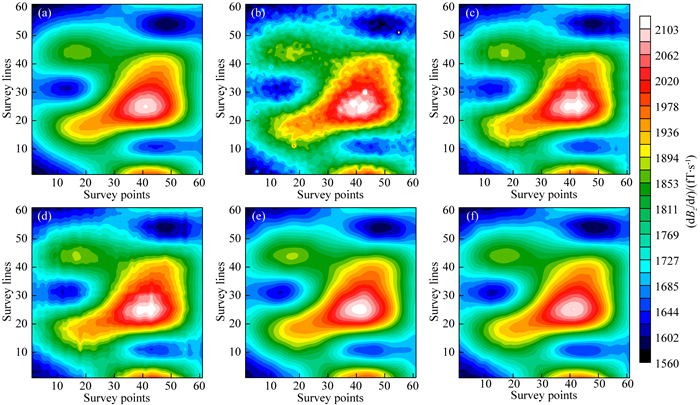图 8 (a) 航空电磁某一时间道理论数据；(b)含噪信号，SNR=40.18 dB；(c) SVD去噪结果，SNR=44.91 dB；(d) PCA去噪结果，SNR=45.19 dB；(e) WT去噪结果，SNR=47.01 dB；(f)曲波变换去噪结果，SNR=50.86 dB. Fig. 8 (a) Theoretical AEM signal; (b) The signal with noise added, SNR=40.18 dB; (c) Result of SVD denoising, SNR=44.91 dB; (d) Result of PCA denoising, SNR=45.19 dB; (e) Result of WT denoising, SNR=47.01 dB; (f) Result of curvelet transform denoising, SNR=50.86 dB

2.2 实测数据去噪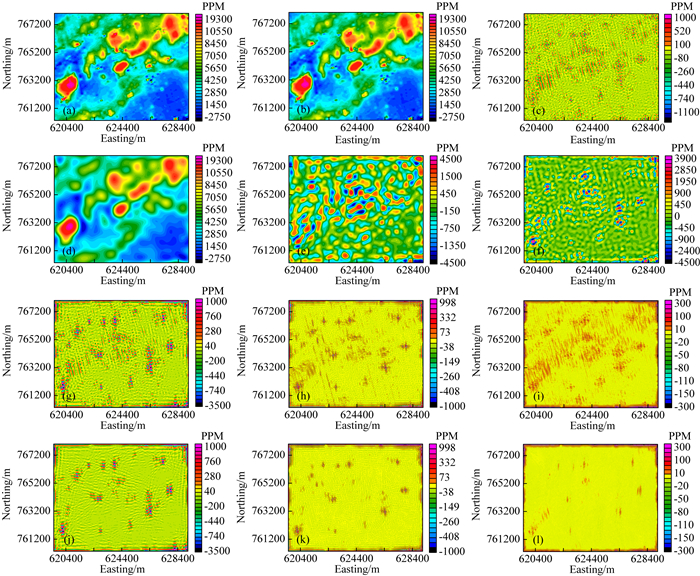图 9 爱尔兰Knock和Weston地区实测数据曲波变换重构及去噪结果 (a)原始观测数据；(b)曲波去噪结果；(c)曲波变换去除的噪声；(d)第一尺度重构；(e)第二尺度重构；(f)第三尺度重构；(g)第四尺度重构；(h)第五尺度重构；(i)第六尺度重构；(j)去噪后第四尺度重构；(k)去噪后第五尺度重构；(l)去噪后第六尺度重构. Fig. 9 Reconstruction and denoising of the survey data from Knock and Weston, Ireland by curvelet transform (a) Original survey data; (b) Result of curvelet denoising; (c) Noise removed by curvelet transform; (d) Reconstruction of 1st scale; (e) Reconstruction of 2nd scale; (f) Reconstruction of the 3rd scale; (g) Reconstruction of 4th scale; (h) Reconstruction of 5th scale; (i) Reconstruction of 6th scale; (j) Reconstruction of 4th scale after denoising; (k) Reconstruction of 5th scale after denoising; (l) Reconstruction of 6th scale after denoising.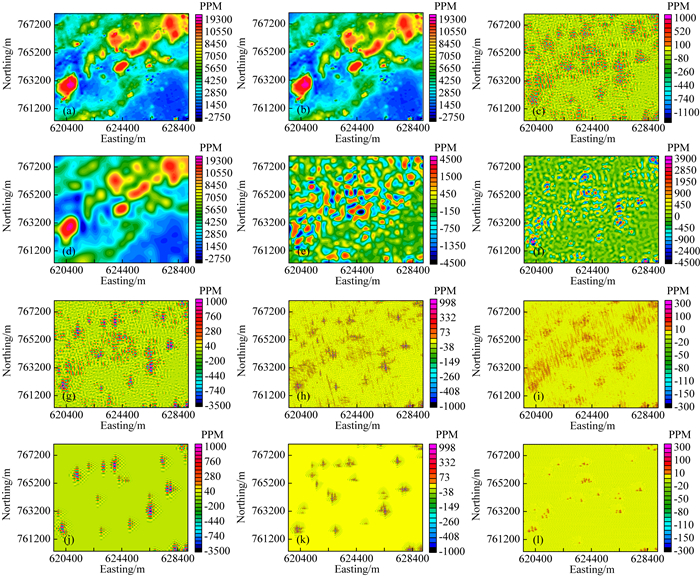图 10 爱尔兰Knock和Weston地区实测数据WT重构及去噪结果 (a)原始观测数据；(b) WT去噪结果；(c) WT去除的噪声；(d)第五尺度近似分量；(e)第五尺度细节分量；(f)第四尺度细节分量；(g)第三尺度细节分量；(h)第二尺度细节分量；(i)第一尺度细节分量；(j)去噪后第三尺度细节分量；(k)去噪后第二尺度细节分量；(l)去噪后第一尺度细节分量. Fig. 10 Reconstruction and denoising of the survey data from Knock and Weston, Ireland by WT method (a) Original survey data; (b) Result of WT denoising; (c) Noise removed by WT; (d) Approximation component of 5th scale; (e) Detailed component of 5th scale; (f) Detailed component of 4th scale; (g) Detailed component of 3rd scale; (h) Detailed component of 2nd scale; (i) Detailed component of 1st scale; (j) Detailed component of 3rd scale after denoising; (k) Detailed component of 2nd scale after denoising; (l) Detailed component of 1st scale after denoising.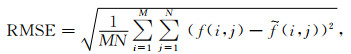(23)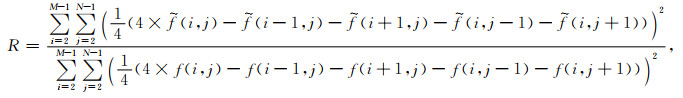(24)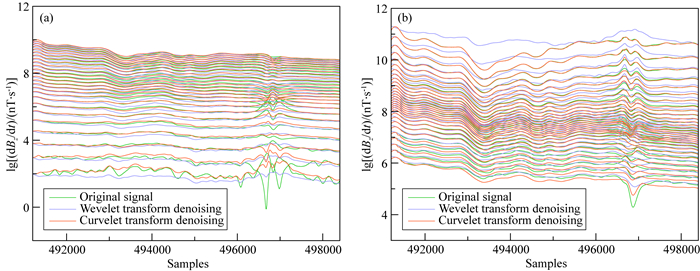图 11 加拿大Iroquois Falls地区实测数据去噪结果 (a)对应于半正弦波的前25时间道数据去噪结果；(b)对应于梯形波的后30时间道数据去噪结果. Fig. 11 Denoising of survey data from Iroquois Falls, Canada (a) Denoising of the first 25 time channels corresponding to the half-sine transmitting wave; (b) Denoising of the last 30 time channels corresponding to the trapezoidal wave.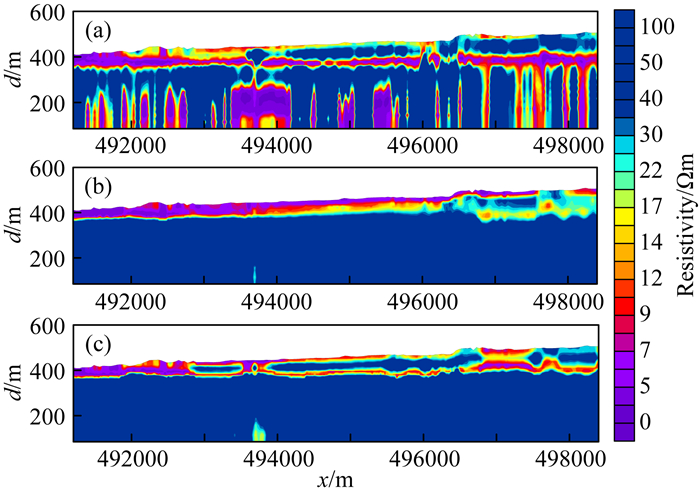图 12 加拿大Iroquois Falls地区实测数据成像结果 (a)原始信号成像结果；(b) WT去噪后信号成像结果；(c)曲波变换去噪后信号成像结果. Fig. 12 Imaging results of survey data from Iroquois Falls, Canada (a) Imaging of original signal; (b) Imaging of WT denoised data; (c) Imaging of curvelet transform denoised data.
3 结论

References
 Abma R, Kabir N. 2006. 3D interpolation of irregular data with a POCS algorithm. Geophysics, 71(5): E91-E97. Candès E, Donoho D L. 1999. Curvelets:A Surprisingly Effective Nonadaptive Representation for Objects with Edges. Nashville: Vanderbilt University Press: 1-10. Candès E, Donoho D L. 2004. New tight frames of curvelets and optimal representations of objects with piecewise C2 singularities. Communications on Pure and Applied Mathematics, 57(2): 219-266. DOI:10.1002/cpa.10116 Candès E, Demanet L, Donoho D, et al. 2006. Fast discrete curvelet transforms. Multiscale Modeling & Simulation, 5(3): 861-899. Cao J J, Yang Z Q, Yang Y, et al. 2018. An adaptive seismic random noise elimination method based on curvelet transform. Geophysical Prospecting for Petroleum (in Chinese), 57(1): 72-78, 121. Donoho D L, Johnstone J M. 1994. Ideal spatial adaptation by wavelet shrinkage. Biometrika, 81(3): 425-455. DOI:10.1093/biomet/81.3.425 Donoho D L. 1995. De-noising by soft-thresholding. IEEE Transactions on Information Theory, 41(3): 613-627. DOI:10.1109/18.382009 Du X Z, Lu C D. 2016-11-09. Singular value decomposition and wavelet analysis-based time-domain aeronautical electromagnetic data denoising method, CN, 106094046A. Herrmann F J, Hennenfent G. 2008. Non-parametric seismic data recovery with curvelet frames. Geophysical Journal International, 173(1): 233-248. DOI:10.1111/j.1365-246X.2007.03698.x Li J W, Liu X B, Zhou J H, et al. 2019. A curvelet threshold iteration method based on energy ratio for surface-wave suppression. Oil Geophysical Prospecting (in Chinese), 54(5): 997-1004. Li L F, Zhang X C, Zhang H, et al. 2015. Feature extraction of non-stochastic surfaces using curvelets. Precision Engineering, 39: 212-219. DOI:10.1016/j.precisioneng.2014.09.003 Qi S H, Liu Q Y, Chen J H, et al. 2016. Attenuation of noise in receiver functions using curvelet transform. Chinese Journal of Geophysics (in Chinese), 59(3): 884-896. DOI:10.6038/cjg20160311 Reninger P A, Martelet G, Deparis J, et al. 2011. Singular value decomposition as a denoising tool for airborne time domain electromagnetic data. Journal of Applied Geophysics, 75(2): 264-276. DOI:10.1016/j.jappgeo.2011.06.034 Ridsdill-Smith T A, Dentith M C. 1999. The wavelet transform in aeromagnetic processing. Geophysics, 64(4): 1003-1013. DOI:10.1190/1.1444609 Sykes M P, Das U C. 1998. Removal of herringbone effects from AEM data maps using the Radon transform. Exploration Geophysics, 29(1-2): 92-95. DOI:10.1071/EG998092 Tao K, Zhu J J. 2012. A comparative study on validity assessment of wavelet de-noising. Journal of Geodesy and Geodynamics (in Chinese), 32(2): 128-133. Wang L P. 2006. Plancherel theorem in L2(Rn). Journal of the Central University for Nationalities (Natural Sciences Edition) (in Chinse), 15(4): 334-338. Ying L X, Demanet L, Candès E. 2005.3D discrete curvelet transform.//Proceedings of SPIE 5914, Wavelets XI. San Diego, California, United States: SPIE, 351-361. Zhang H L, Liu T Y, Zhang Y C. 2010. High order correlation based dip angle scanning noise elimination method in curvelet domain and space domain. Oil Geophysical Prospecting (in Chinese), 45(2): 208-214. Zhu K G, Wang L Q, Xie B, et al. 2013. Noise removal for airborne electromagnetic data based on principal component analysis. The Chinese Journal of Nonferrous Metals (in Chinese), 23(9): 2430-2435. 曹静杰, 杨志权, 杨勇, 等. 2018. 一种基于曲波变换的自适应地震随机噪声消除方法. 石油物探, 57(1): 72-78, 121. 杜兴忠, 陆从德. 2016-11-09.基于奇异值分解和小波分析的时间域航空电磁数据去噪方法.中国, 106094046A. 李继伟, 刘晓兵, 周俊骅, 等. 2019. 基于能量比的Curvelet阈值迭代面波压制. 石油地球物理勘探, 54(5): 997-1004. 齐少华, 刘启元, 陈九辉, 等. 2016. 接收函数的曲波变换去噪. 地球物理学报, 59(3): 884-896. DOI:10.6038/cjg20160311 陶珂, 朱建军. 2012. 小波去噪质量评价方法的对比研究. 大地测量与地球动力学, 32(2): 128-133. 王丽萍. 2006. 平方可测空间的Plancherel定理. 中央民族大学学报(自然科学版), 15(4): 334-338. 张恒磊, 刘天佑, 张云翠. 2010. 基于高阶相关的Curvelet域和空间域的倾角扫描噪声压制方法. 石油地球物理勘探, 45(2): 208-214. 朱凯光, 王凌群, 谢宾, 等. 2013. 基于主成分分析的航空电磁数据噪声去除方法. 中国有色金属学报, 23(9): 2430-2435.